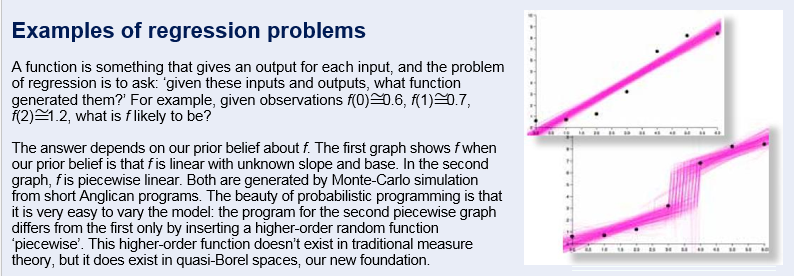## Complex statistical methods benefit from probabilistic programming

New ways of understanding and building basic structures in statistics and machine learning is the research area of Professor Sam Staton, a Royal Society University Research Fellow. In this article he explains his work into understanding what a probabilistic program means as a mathematical structure.

Probabilistic programming is emerging as a way of building the kinds of complex statistical models that are used in computational statistics and machine learning. Businesses from Uber to YouGov are investing in it. In brief, a probabilistic program is a program that uses two additional programming features: one for making random choices, and one for recording observations about data. What makes it a statistical model is the idea that a probabilistic program will not simply be run once. Rather it will be treated as a model specification, and typically run thousands of times, so that all the random choices are considered: this is called a ‘Monte Carlo’ simulation.

For example, a simple 'Bayesian' inference problem involves updating a 'prior' belief according to the likelihood of some observation. This can be coded up as a two-line probabilistic program, in which the first line makes a random choice (incorporating the prior belief) and the second records an observation (incorporating the likelihood). We find the updated 'posterior' belief by running a Monte Carlo simulation.

The interest in this method of statistical modelling comes from the separation between the model (now a program) and the simulation method. Some simulation methods are very efficient and yet they can be applied to a wide variety of programs. This makes it easy to prototype different models without rewriting new simulation methods each time.

My own work in this area (with colleagues in Oxford and elsewhere) is based around understanding the meaning of probabilistic programs. One can always run programs and get results, but I’d argue that it is important to understand what a program means as a mathematical structure. This general point of view was first emphasised in Oxford 50 years ago by Dana Scott and Christopher Strachey. The conventional foundation for probability theory is called measure theory. It turns out that while the measure-theoretic interpretation of probabilistic programs is often quite elegant, there are some useful probabilistic programs that are taxing for this traditional foundation.

As an example, let's consider higher order random functions - random functions that themselves produce random functions. In conventional software engineering, higher order functions are an important way of building modular programs. Probabilistic programming systems, such as the Anglican system developed in Oxford, have no problem with higher-order functions in practice. However, the traditional measure-theoretic foundation of statistics cannot cope with higher-order functions: this was proved by Aumann in 1961. Our new direction in measure theory, based on ‘quasi-Borel spaces’, can cope with higher-order functions, and so it is a good foundation for probabilistic programming.

This is what I find exciting about my research: that structural analysis from logic and programming can suggest new ways of understanding and building basic structures in statistics and machine learning.